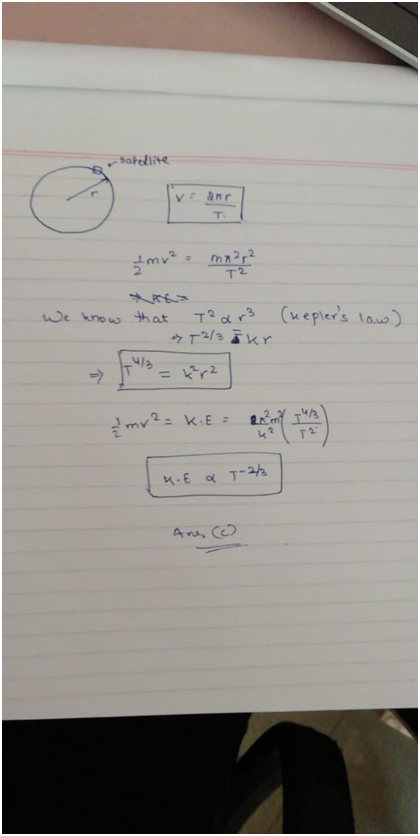Click to Chat

1800-1023-196

+91-120-4616500

CART 0

• 0

MY CART (5)

Use Coupon: CART20 and get 20% off on all online Study Material

ITEM
DETAILS
MRP
DISCOUNT
FINAL PRICE
Total Price: Rs.

There are no items in this cart.
Continue Shopping
```
If the time period of a satellite is T, its kinetic energy is proportional to KE directly proportional to T -1/2 KE directly proportional to T -2/3 KE directly proportional to T 3/2 d.None of these

```
one year ago

```							Answer of the question is C.The solution of the question is the given in the image below.I hope it helps!!```
one year ago
```							Let KE = xCentripetal force = C= mv²/r Gravitational force:= g=GMm/r² => C:g= mv²/r : GMm/r²  ⇒v² = GM/r  => x = ½m(GM/r) = GM/2r  Here this shows that x(kinetic energy of the satellite) is inversely proportional to the radius of the satellite orbit: ⇒x ∝ r ⁻¹  We have time of revolution given as: T = 2π/ω  Centripetal Force= FcGravitational Force= FgFc = Fg  mv²/r = GMm/r²m(ωr)²/r = GMm/r²  m(ω²)r = GMm/r²  ω² = GM/r³  Now we have,T²= 4π²/ω² T²=4π²/(GM/r³) = 4π²r³/GM  The above equation shows that the square of the time period is proportional to the cube  of the radius such that: T² ∝ r³ (also known as Kepler's 3rd Law) => T ∝ r^3/2  If T = c1*r^3/2 where we hace c1 as some constant, and r = c2*x⁻¹, then:  T = c1*(c2x⁻¹)^3/2 = (c1*(c2^3/2))*x^-3/2 = c3*x^-3/2, thus we achieve the following relation of time period revolution:⇒ T ∝ x^-3/2 so KE ∝ T-2/3
```
one year ago
Think You Can Provide A Better Answer ?

## Other Related Questions on General Physics

View all Questions »### Course Features

• 731 Video Lectures
• Revision Notes
• Previous Year Papers
• Mind Map
• Study Planner
• NCERT Solutions
• Discussion Forum
• Test paper with Video Solution### Course Features

• 18 Video Lectures
• Revision Notes
• Test paper with Video Solution
• Mind Map
• Study Planner
• NCERT Solutions
• Discussion Forum
• Previous Year Exam Questions